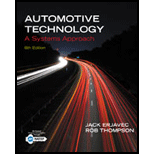Chapter 17, Problem 2ASRQAutomotive Technology: A Systems A...

6th Edition
Jack Erjavec + 1 other
ISBN: 9781133612315

Solutions

Chapter
SectionAutomotive Technology: A Systems A...

6th Edition
Jack Erjavec + 1 other
ISBN: 9781133612315
Textbook Problem

Technician A says that the reserve rating of a battery is the amount of steady current that a fully charged battery can supply tor 20 hours without the voltage falling below 10.5 volts. Technician B says that ampere-hour ratings state how many hours the battery’ is capable of supplying 25 amperes. Who is correct? Technician A Technician BBoth A and BNeither A nor B

To determine

The technician with correct approach.

Explanation

Given:

Technician A says that the reserve rating of a battery is the amount of steady current that a fully charged battery can supply for 20 hours without the voltage falling below 10.5 volts.

Technician B says that ampere-hour ratings state how many hours the battery is capable of supplying 25 amperes...

Still sussing out bartleby?

Check out a sample textbook solution.

See a sample solution

The Solution to Your Study Problems

Bartleby provides explanations to thousands of textbook problems written by our experts, many with advanced degrees!

Get Started

Calculate tailstock setover for a 13.5" part with a TPF of 0.27.

Precision Machining Technology (MindTap Course List)

What are the basic characteristics of a NoSQL database?

Database Systems: Design, Implementation, & Management

What do we mean by the determinant of a matrix?

Engineering Fundamentals: An Introduction to Engineering (MindTap Course List)

Find the rectangular representation of the force F, given that its magnitude is 320 N.

International Edition---engineering Mechanics: Statics, 4th Edition

Define a use case and a use case diagram, and prepare a sample of each.

Systems Analysis and Design (Shelly Cashman Series) (MindTap Course List)